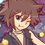# Geometry (Thailand Math POSN 1st elimination round 2014)

A lot easier than last year.

Write a full solution.

1.) Let $\square ABCD$ be a quadrilateral with the sum of the opposite sides are equal. (i.e. $AB + CD = AD + BC$). Prove that

• 1.1) $\square ABCD$ is a tangential quadrilateral (there is a circle inside the square and tangent to each sides).
• 1.2) The 4 internal angle bisectors intersect at one point.

2.) Let $\triangle ABC$ be the triangle with point $D$ lies on $\overline{BC}$ such that $AB\times DC = AC \times BD$. Prove that $\overline{AD}$ is the internal angle bisector of $B\hat{A}C$.

3.) Let $\triangle ABC$ be the triangle with $B\hat{A}C < 90^{\circ}$ and $BC = a, CA = b, AB = c$. If $\overline{AD}$ is a median line, prove that $\displaystyle 2(AD^{2}) = b^{2}+c^{2}-\frac{a^{2}}{2}$.

4.) Given a line segment length $1$ unit. Explain how to construct a square with area of $5\sqrt{3}$ sq.unit using only straightedge and compass.

5.) Let the external angle bisectors of $\triangle ABC$ bisect at the extension of sides of triangle at $D,E,F$. Prove that $D,E,F$ are collinear.

Check out all my notes and stuffs for more problems!

Thailand Math POSN 2013

Thailand Math POSN 2014Note by Samuraiwarm Tsunayoshi
6 years, 9 months ago

This discussion board is a place to discuss our Daily Challenges and the math and science related to those challenges. Explanations are more than just a solution — they should explain the steps and thinking strategies that you used to obtain the solution. Comments should further the discussion of math and science.

When posting on Brilliant:

• Use the emojis to react to an explanation, whether you're congratulating a job well done , or just really confused .
• Ask specific questions about the challenge or the steps in somebody's explanation. Well-posed questions can add a lot to the discussion, but posting "I don't understand!" doesn't help anyone.
• Try to contribute something new to the discussion, whether it is an extension, generalization or other idea related to the challenge.

MarkdownAppears as
*italics* or _italics_ italics
**bold** or __bold__ bold
- bulleted- list
• bulleted
• list
1. numbered2. list
1. numbered
2. list
Note: you must add a full line of space before and after lists for them to show up correctly
paragraph 1paragraph 2

paragraph 1

paragraph 2

[example link](https://brilliant.org)example link
> This is a quote
This is a quote
    # I indented these lines
# 4 spaces, and now they show
# up as a code block.

print "hello world"
# I indented these lines
# 4 spaces, and now they show
# up as a code block.

print "hello world"
MathAppears as
Remember to wrap math in $$ ... $$ or $ ... $ to ensure proper formatting.
2 \times 3 $2 \times 3$
2^{34} $2^{34}$
a_{i-1} $a_{i-1}$
\frac{2}{3} $\frac{2}{3}$
\sqrt{2} $\sqrt{2}$
\sum_{i=1}^3 $\sum_{i=1}^3$
\sin \theta $\sin \theta$
\boxed{123} $\boxed{123}$

Sort by:

For q3 will you get marks if you quote Apollonius' Theorem? It is quite well known.

- 6 years, 9 months ago

Nah, but it's actually really easy to prove.

- 6 years, 9 months ago